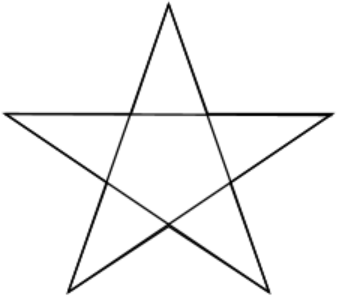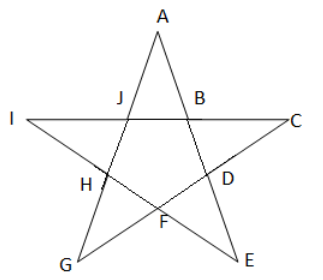Courses
Courses for Kids
Free study material
Free LIVE classes
MoreLIVE
Join Vedantu’s FREE Mastercalss

# How many triangles are there in the diagram given below?A. 6B. 10C. 12D. 14Verified
334.8k+ views
Hint: We will first name all of the vertices of the star and count vertex by vertex how many triangles are there and this will give us our answer.

Complete step-by-step solution:From the figure, we can see that A, C, E, G, and I are the outer vertices of the figure, and B, D, F, H, and J are the inner vertices of the figure.
Now, starting from vertex A, we can see that there are a total of 5 small triangles which have one vertex as an out vertex of the figure and the other two vertices as the inner vertices of the figure. They are given as:
\begin{align} & \Delta AJB \\ & \Delta CBD \\ & \Delta EDF \\ & \Delta GFH \\ & \Delta IJH \\ \end{align}
Therefore, there are 5 small triangles in the given figure.
Now we will count the big triangles in the figure.
Starting from vertex J, we can see that there are also 5 bigger triangles in this figure which have one vertex as an inner vertex of the figure and the other two vertices as the outer vertices of the figure. They are given as:
\begin{align} & \Delta JCG \\ & \Delta BEI \\ & \Delta DGA \\ & \Delta FIC \\ & \Delta HAE \\ \end{align}
Therefore, there are 5 big triangles in the given figure.
Thus, the total number of triangles in the given figure is:
$\Rightarrow 5+5=10$
Therefore, there are a total of 10 triangles in figure (5 small and 5 big).
Hence, option (B) is the correct option.

Note: Don’t forget to name the vertices as it is very important in this type of question. Always name the vertices of the figure and count the triangles in reference to the unique vertex. It decreases the scope of mistakes and results in the most accurate answer.

Last updated date: 25th Sep 2023
Total views: 334.8k
Views today: 10.34k